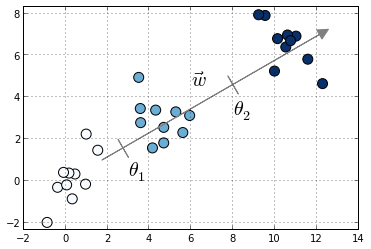# mord: Ordinal Regression in Python¶

mord is a Python package that implements some ordinal regression methods following the scikit-learn API.## What is ordinal regression ?¶

Ordinal Regression denotes a family of statistical learning methods in which the goal is to predict a variable which is discrete and ordered. For example, predicting the movie rating on a scale of 1 to 5 starts can be considered an ordinal regression task.

In this package we provide different models for the ordinal regression task. We categorize them between Threshold-based models, Regression-based models and Classification-based models.

# Citing¶

If you find this software useful, please consider citing:

Fabian Pedregosa-Izquierdo. Feature extraction and supervised learning on fMRI: from practice to theory. PhD thesis.